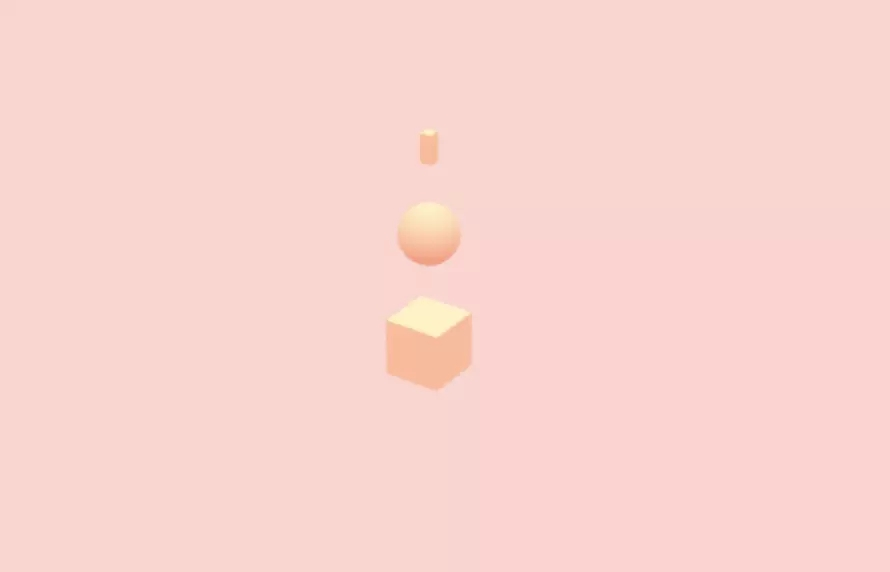# 怎么在Three.js中使用矩阵和向量

```var box_geometry = new THREE.BoxGeometry();
var sphere_geometry = new THREE.SphereGeometry(0.5, 32, 32);
var cylinder_geometry = new THREE.CylinderGeometry(0.1, 0.1, 0.5);

var material = new THREE.MeshLambertMaterial({color: new THREE.Color(0.9, 0.55, 0.4)})```

```var box = new THREE.Mesh(box_geometry, material);
var sphere = new THREE.Mesh(sphere_geometry, material);
sphere.position.y += 1;
var cylinder = new THREE.Mesh(cylinder_geometry, material);
cylinder.position.y += 1.75;
scene.add(box);
scene.add(sphere);
scene.add(cylinder);``````box.scale.multiplyScalar(0.5);
sphere.scale.multiplyScalar(0.5);
cylinder.scale.multiplyScalar(0.5);``````var pile = new THREE.Object3D();
pile.scale.multiplyScalar(0.5);
pile.add(box);
pile.add(sphere);
pile.add(cylinder);
scene.add(pile);``````box.matrixAutoUpdate = false;
sphere.matrixAutoUpdate = false;
cylinder.matrixAutoUpdate = false;```

```var sphere_matrix = new THREE.Matrix4().makeTranslation(0.0, 1.0, 0.0);
sphere.applyMatrix(sphere_matrix);
var cylinder_matrix = sphere_matrix.clone(); cylinder_matrix.multiply(new THREE.Matrix4().makeTranslation(0.0, 0.75, 0.0));
cylinder.applyMatrix(cylinder_matrix);````sphere_matrix.multiply(new THREE.Matrix4().makeRotationZ(-Math.PI * 0.25));`Three.js中最常见的一种4x4的矩阵，被称为变换矩阵，它所表示的变换类型包括平移、旋转和缩放。```t = |1 0 0 10|
|0 1 0 40|
|0 0 1 0 |
|0 0 0 1 |```

```|20| |1 0 0 10| |30|
|20| x |0 1 0 40| = |60|
|0 | |0 0 1 0 | |0 |
|1 | |0 0 0 1 | |1 |```

transformedVector = vector * transformationMatrix

```var vector = new THREE.Vector3(20, 20, 0);
var matrix = new THREE.Matrix4();
matrix.makeTranslation(10, 40, 0);
vector.applyMatrix4(matrix);```

```matrix.makeRotationX(angle);
matrix.makeRotationY(angle);
matrix.makeRotationZ(angle);
matrix.makeRotationAxis(axis, angle);
matrix.makeRotationFromEuler(euler);
matrix.makeRotationFromQuaternion(quaternion);```

```matrix.makeRotationX(Math.PI);
matrix.makeRotationAxis(new THREE.Vector3(1, 0, 0), Math.PI);```

```var translation = new THREE.Vector3();
var rotation = new THREE.Quaternion();
var scale = new THREE.Vector3();
var matrix = new THREE.Matrix4();
matrix.compose(translation, rotation, scale);````combinedMatrix = rotationMatrix * scaleMatrix * translationMatrix;`

Three.js里提供了两种矩阵相乘的方法：

• matrix.multiply(otherMatrix)

• matrix.multiplyMatrices(matrixA, matrixB)

```4 x 5 = 20
20 / 5 = 4```

Three.js里提供了一种计算逆矩阵的方法：

```var matrix = new THREE.Matrix4();
var inverseMatrix = new THREE.Matrix4();
matrix.getInverse(inverseMatrix);```